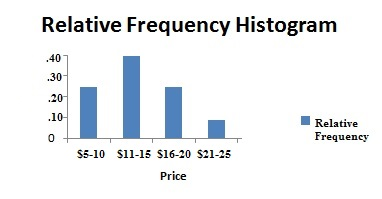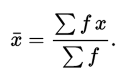# Grouped Data / Ungrouped Data: Definition, Examples

Share on

Contents:

Watch the video for an overview and how to find the mean of grouped data:

## What is Grouped Data?

Grouped data is data that has been bundled together in categories. Histograms and frequency tables can be used to show this type of data:Relative frequency histogram showing book sales for a certain day, sorted by price.

The data is grouped together by classes or bins.

## Grouped vs. Ungrouped Data

Ungrouped data is the data you first gather from an experiment or study. The data is raw — that is, it’s not sorted into categories, classified, or otherwise grouped. An ungrouped set of data is basically a list of numbers.

## Calculating the Sample Mean for Grouped Data

When you have a frequency table or other group of data, the original set of data is lost — replaced with statistics for the group. You can’t find the exact sample mean (as you don’t have the original data) but you can find an estimate. The formula for estimating the sample mean for data that has been grouped is:• x̄ is the sample mean,
• x is the class (or category) midpoint,
• f is the class frequency.
• Example question: Find the sample mean for the following frequency table.

Score Frequency ( f )
Between 5 and 10 1
10 ≤ t < 15 4
15 ≤ t < 20 6
20 ≤ t < 25 4
25 ≤ t < 30 2
30 ≤ t < 35 3
TOTALS 20

Step 1: Find the midpoint for each class interval. the midpoint is just the middle of each interval. For example, the middle of 10 and 15 is 12.5:

Score Frequency ( f ) Midpoint ( x )
Between 5 and 10 1 7.5
10 ≤ t < 15 4 12.5
15 ≤ t < 20 6 17.5
20 ≤ t < 25 4 22.5
25 ≤ t < 30 2 27.5
30 ≤ t < 35 3 32.5
TOTALS 20

Step 2: Multiply the midpoint (x) by the frequency (f):

Score Frequency ( f ) Midpoint ( x ) Midpoint x * frequency f
Between 5 and 10 1 7.5 7.5
10 ≤ t < 15 4 12.5 50
15 ≤ t < 20 6 17.5 105
20 ≤ t < 25 4 22.5 90
25 ≤ t < 30 2 27.5 55
30 ≤ t < 35 3 32.5 97.5
TOTALS 20 405

Add up all of the totals for this step. In other words, add up all the values in the last column (you should get 405).

Step 3: Divide the last column (f*x) by the second column (f):
The mean (x̄) = 405 / 20 = 20.25.

## References

Agresti A. (1990) Categorical Data Analysis. John Wiley and Sons, New York.
Klein, G. (2013). The Cartoon Introduction to Statistics. Hill & Wamg.

CITE THIS AS:
Stephanie Glen. "Grouped Data / Ungrouped Data: Definition, Examples" From StatisticsHowTo.com: Elementary Statistics for the rest of us! https://www.statisticshowto.com/probability-and-statistics/descriptive-statistics/grouped-data/
---------------------------------------------------------------------------Need help with a homework or test question? With Chegg Study, you can get step-by-step solutions to your questions from an expert in the field. Your first 30 minutes with a Chegg tutor is free!